• 利利用用python绘绘制制中中国国地地图图 含含省省界界河河流流等等 这篇文章主要介绍了利用python绘制中国地图帮助大家更好的理解和使用python绘制图形感兴趣的朋友可 了解下 我们可 使用Basemap这个工具包来实现...
• 主要介绍了利用python绘制中国地图，帮助大家更好的理解和使用python绘制图形，感兴趣的朋友可以了解下
• 我们可以使用Basemap这个工具包来实现中国地图绘制首先需要加载一些包：import numpy as npimport matplotlib.pyplot as pltfrom mpl_toolkits.basemap import BasemapBasemap包就是气象画图的利器，现在我们就...
我们可以使用Basemap这个工具包来实现中国地图的绘制首先需要加载一些包：import numpy as npimport matplotlib.pyplot as pltfrom mpl_toolkits.basemap import BasemapBasemap包就是气象画图的利器，现在我们就可以愉快的画图了！plt.figure(1)map=Basemap()map.drawcoastlines()plt.title(r'$World\ Map$',fontsize=24)plt.show()第2行创建一个地图，第3行添加海岸线，这样一个世界地图就出来了，怎么样，很简单吧。(plt.show()这行代码是用来显示图片的)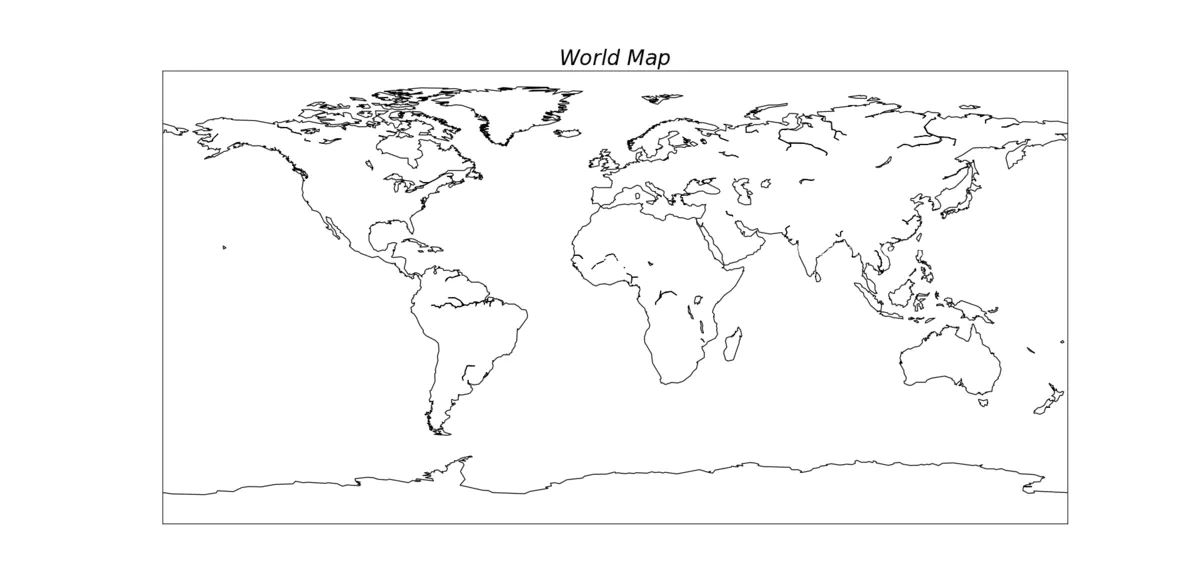我们发现这只是海岸线图，那么怎么将国界线添加上去呢？很简单，只要添加一行代码就可以了。map.drawcountries()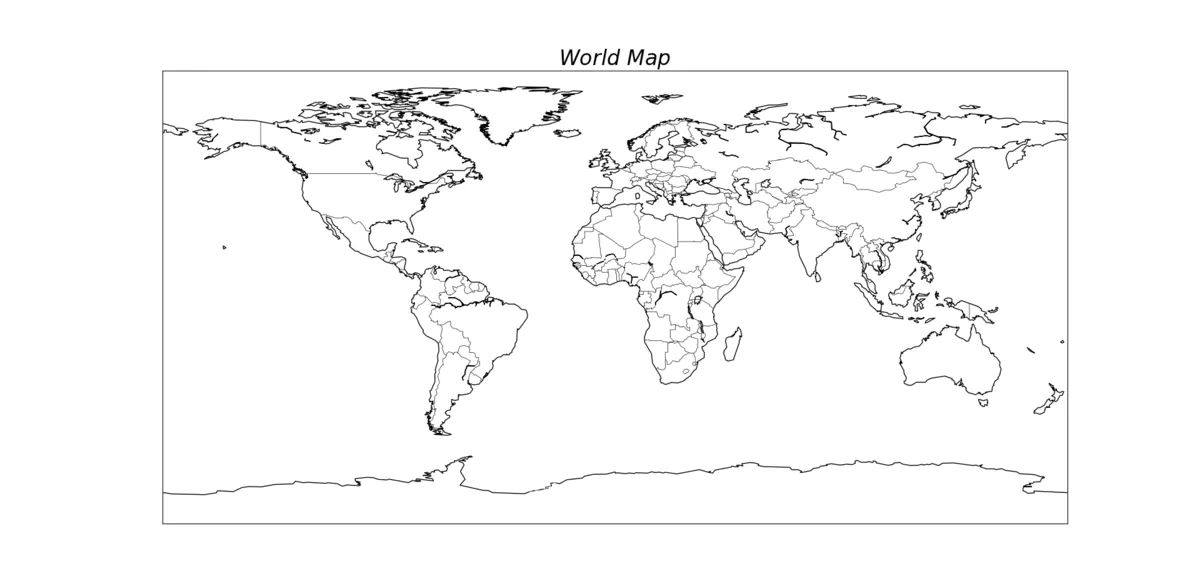那么怎么添加河流呢？可能有些同学已经猜到了，就是drawrivers()map.drawrivers(color='blue',linewidth=0.3)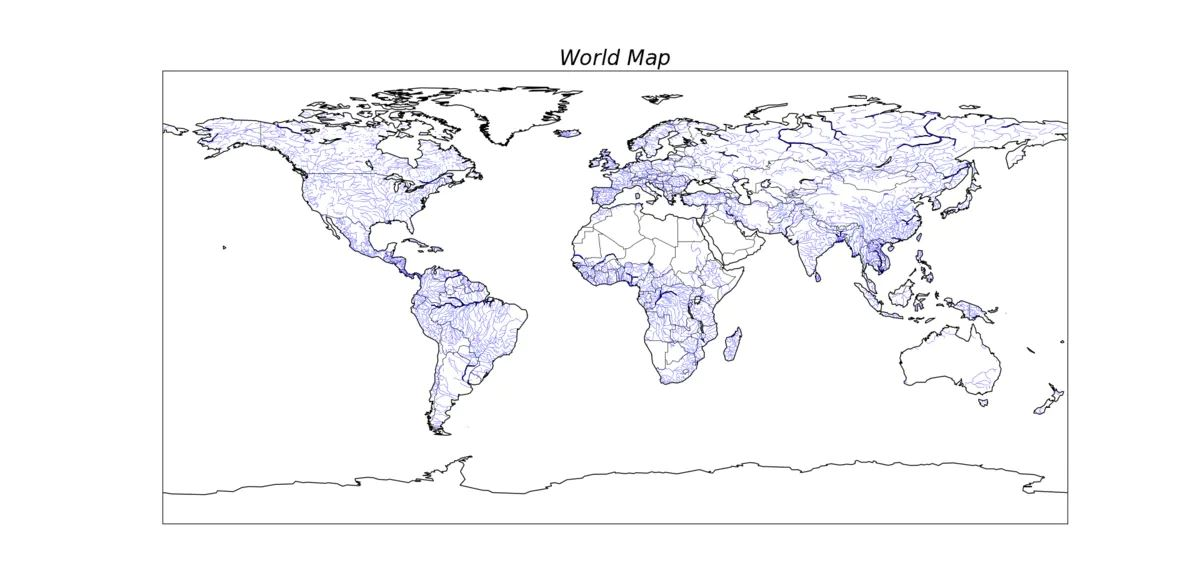好了，现在我们可以开始画中国地图了！其实只要在创建地图时指定一下范围就可以了，查阅资料发现，中国的经纬度范围是东经135度2分30秒-东经73度40分，北纬3度52分-北纬53度33分。map=Basemap(llcrnrlon=70,llcrnrlat=3,urcrnrlon=139,urcrnrlat=54)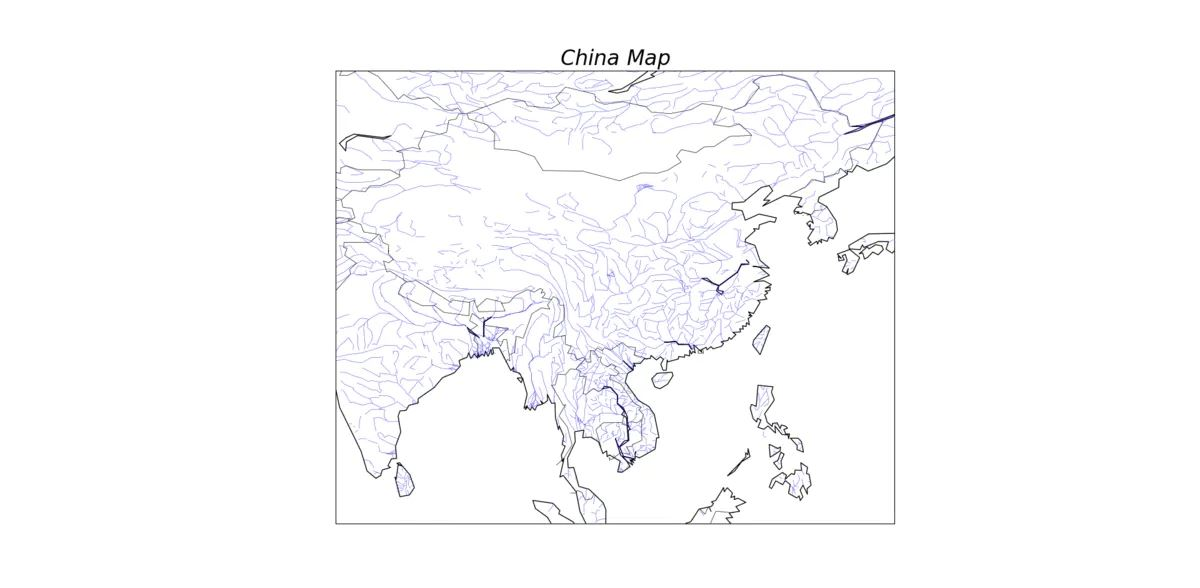好了，一个中国地图就出来了！但是我们发现，好像少了点什么，没错就是省界。我们可以在https://gadm.org/download_country_v3.html下载中国大陆和台湾省的行政区域的shape文件，下载后解压，然后加入下面的代码。CHN='G:\python_material\MapOfChina'CHN的值就是解压后的地图文件所在的地址。下面我们就可以加入省界了！map.readshapefile(CHN+'\gadm36_CHN_shp\gadm36_CHN_1','states',drawbounds=True)别忘了把台湾省加上去map.readshapefile(CHN+'\gadm36_TWN_shp\gadm36_TWN_1','taiwan',drawbounds=True)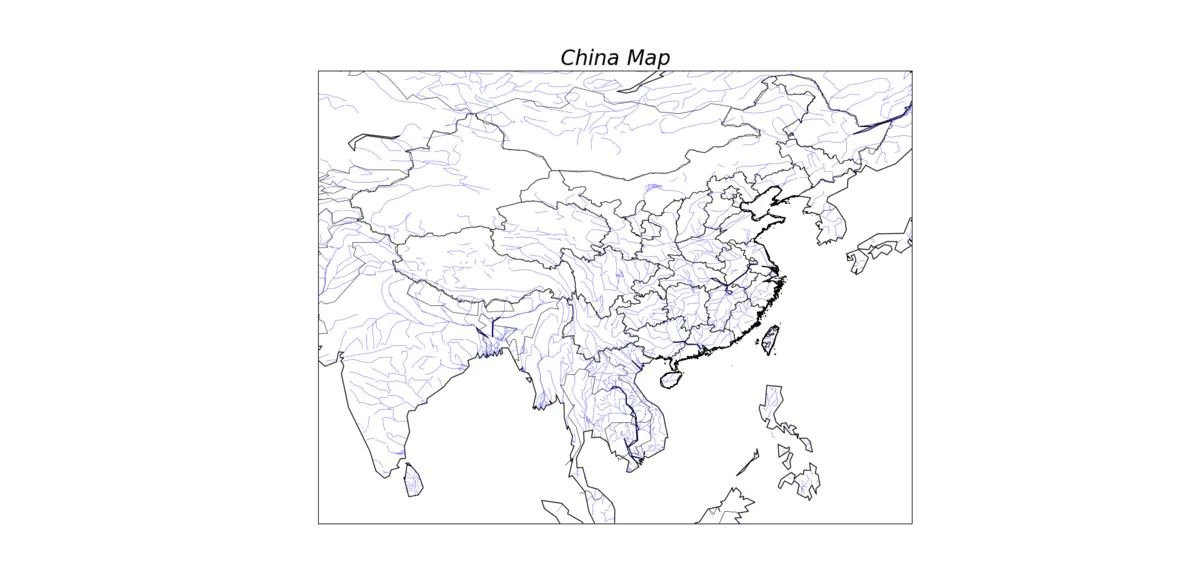还可以在地图上加上经纬度，比如我们要画5条经纬线，可以这么做：parallels = np.linspace(3,55,5)map.drawparallels(parallels,labels=[True,False,False,False])meridians = np.linspace(70,140,5)map.drawmeridians(meridians,labels=[False,False,False,True])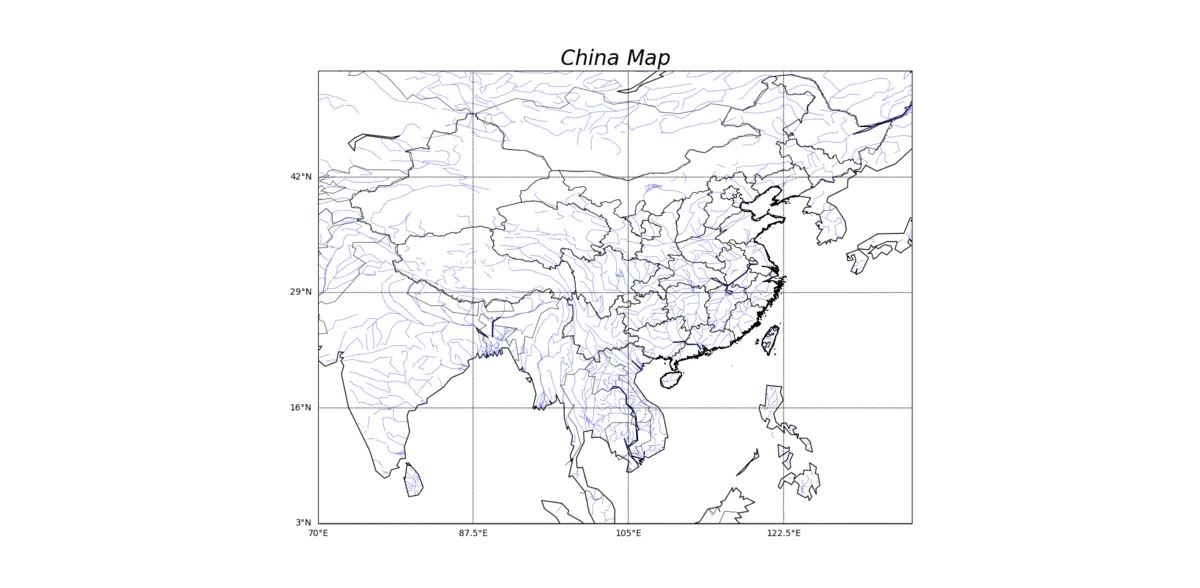大功告成！但是，emmm，我们发现好像有点歪？我们可以在创建地图时选择投影参数。map=Basemap(llcrnrlon=70,llcrnrlat=3,urcrnrlon=137,urcrnrlat=54,projection = 'lcc', lat_1 = 33, lat_2 = 45, lon_0 = 100)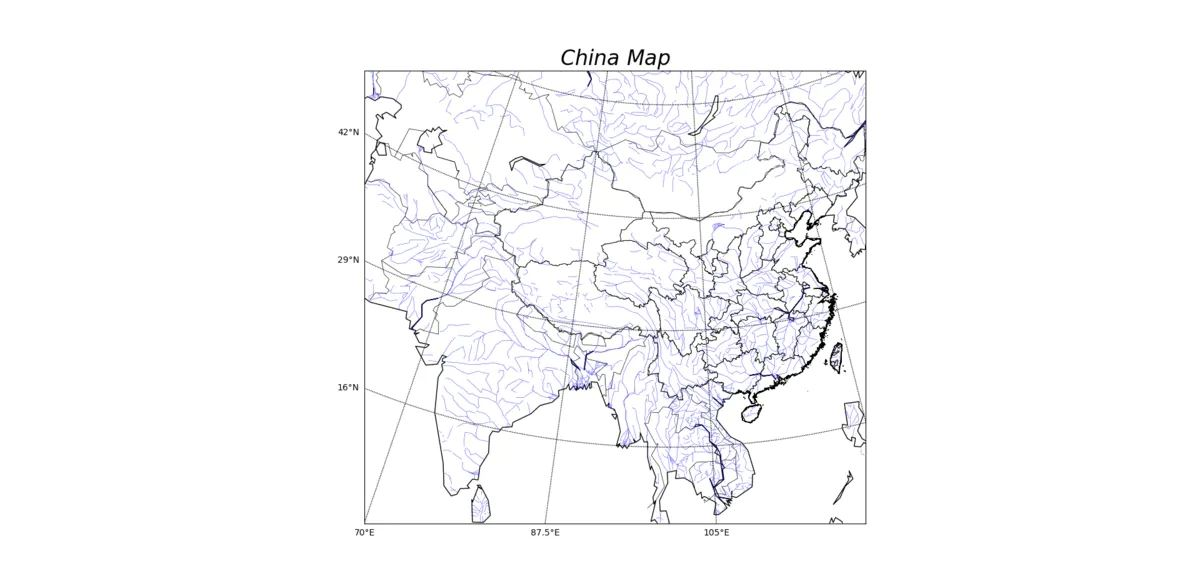这回正式的完成了！附上所有代码：import numpy as npimport matplotlib.pyplot as pltfrom mpl_toolkits.basemap import Basemapplt.figure(1)map=Basemap(llcrnrlon=70,llcrnrlat=3,urcrnrlon=139,urcrnrlat=54,projection = 'lcc', lat_1 = 33, lat_2 = 45, lon_0 = 100)map.drawcoastlines()map.drawcountries()map.drawrivers(color='blue',linewidth=0.3)CHN='G:\python_material\MapOfChina'map.readshapefile(CHN+'\gadm36_CHN_shp\gadm36_CHN_1','states',drawbounds=True)map.readshapefile(CHN+'\gadm36_TWN_shp\gadm36_TWN_1','taiwan',drawbounds=True)parallels = np.linspace(3,55,5)map.drawparallels(parallels,labels=[True,False,False,False])meridians = np.linspace(70,140,5)map.drawmeridians(meridians,labels=[False,False,False,True])plt.title(r'$China\ Map$',fontsize=24)plt.show()以上就是利用python绘制中国地图的详细内容，更多关于python 绘制地图的资料请关注聚米学院其它相关文章！
展开全文• 我们旨在绘制河流塑料污染的地图。 这是一个热门话题，原因如下： 在气候变化之后，塑料污染是对我们海洋的第二大威胁。 海洋塑料污染主要来自陆地。 河流是垃圾进入海洋的通道。 例如，在巴黎市丢失的一瓶酒可能...
• 我们可以使用basemap这个工具包来实现中国地图绘制首先需要加载一些包：import numpy as npimport matplotlib.pyplot as pltfrom mpl_toolkits.basemap import basemapbasemap包就是气象画图的利器，现在我们就...
我们可以使用basemap这个工具包来实现中国地图的绘制首先需要加载一些包：import numpy as npimport matplotlib.pyplot as pltfrom mpl_toolkits.basemap import basemapbasemap包就是气象画图的利器，现在我们就可以愉快的画图了！plt.figure(1)map=basemap()map.drawcoastlines()plt.title(r'$world\ map$',fontsize=24)plt.show()第2行创建一个地图，第3行添加海岸线，这样一个世界地图就出来了，怎么样，很简单吧。(plt.show()这行代码是用来显示图片的)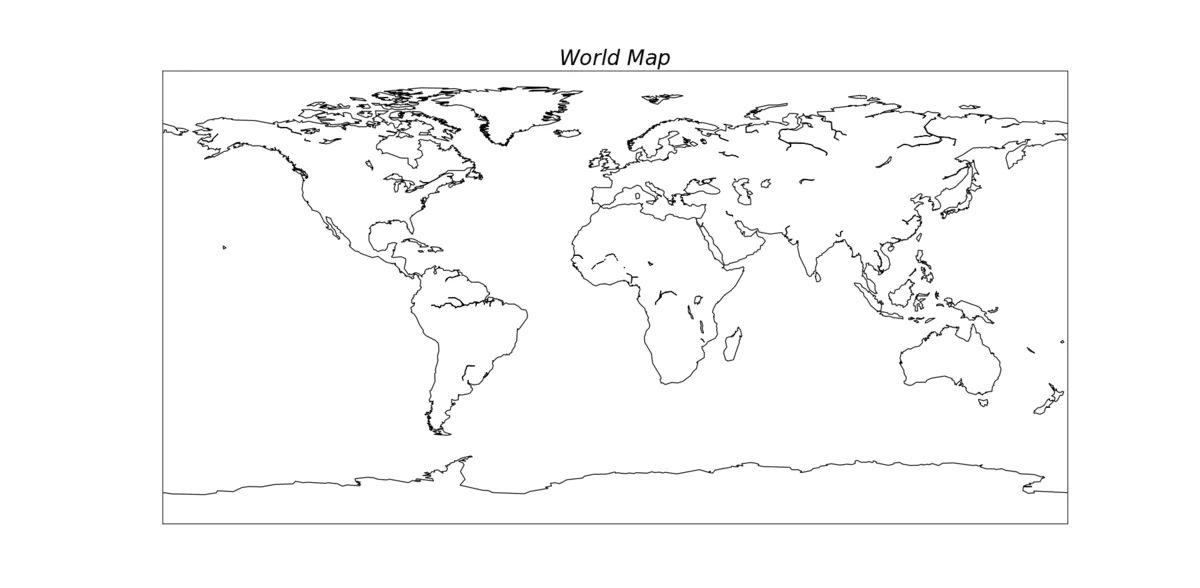我们发现这只是海岸线图，那么怎么将国界线添加上去呢？很简单，只要添加一行代码就可以了。map.drawcountries()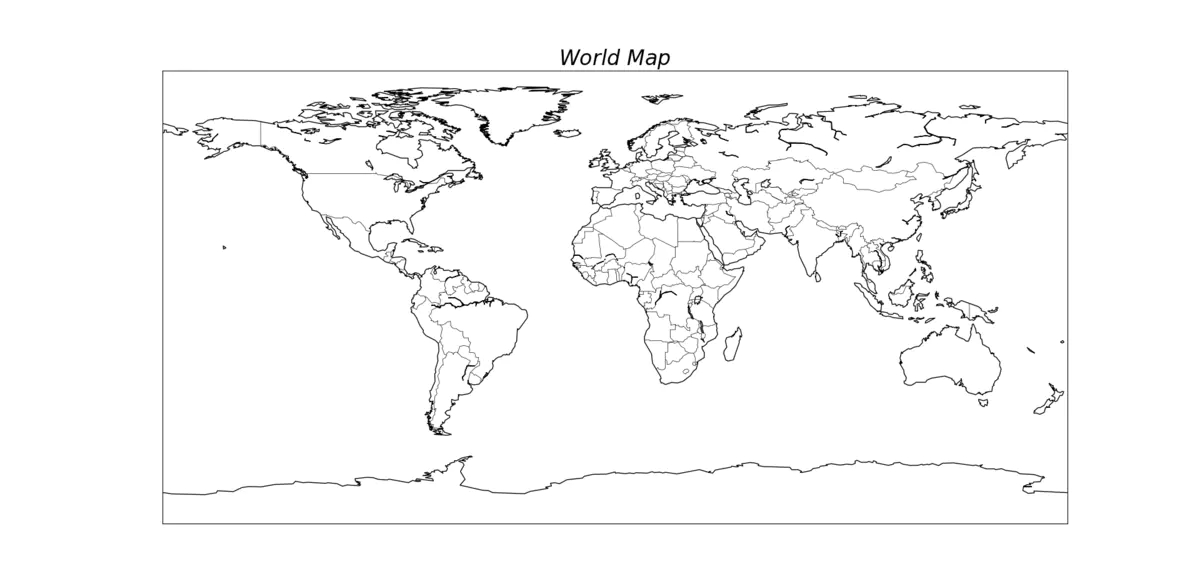那么怎么添加河流呢？可能有些同学已经猜到了，就是drawrivers()map.drawrivers(color='blue',linewidth=0.3)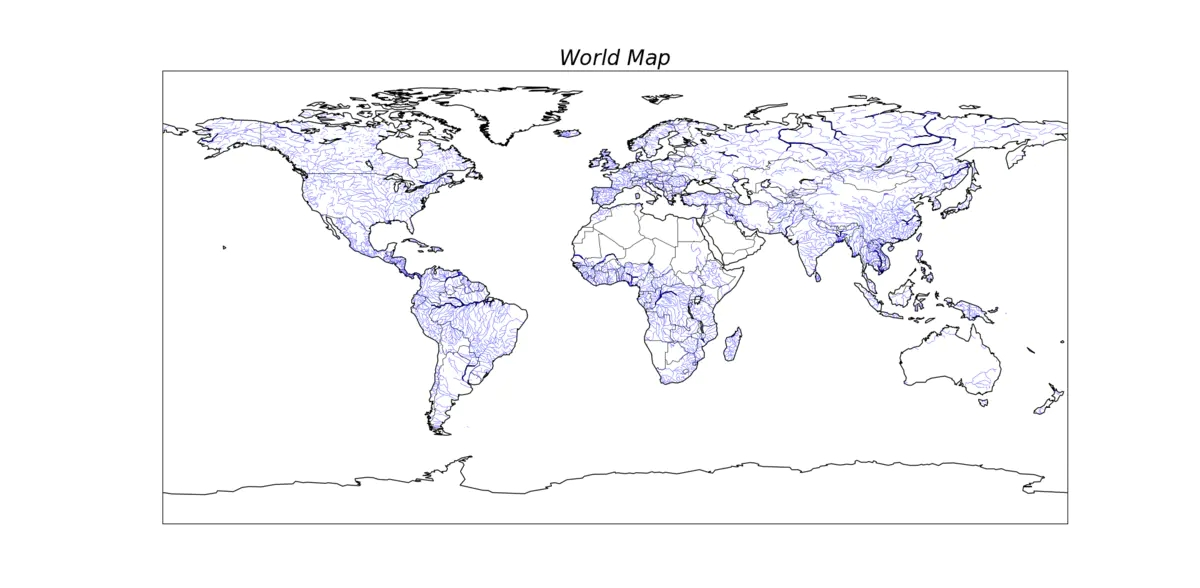好了，现在我们可以开始画中国地图了！其实只要在创建地图时指定一下范围就可以了，查阅资料发现，中国的经纬度范围是东经135度2分30秒-东经73度40分，北纬3度52分-北纬53度33分。map=basemap(llcrnrlon=70,llcrnrlat=3,urcrnrlon=139,urcrnrlat=54)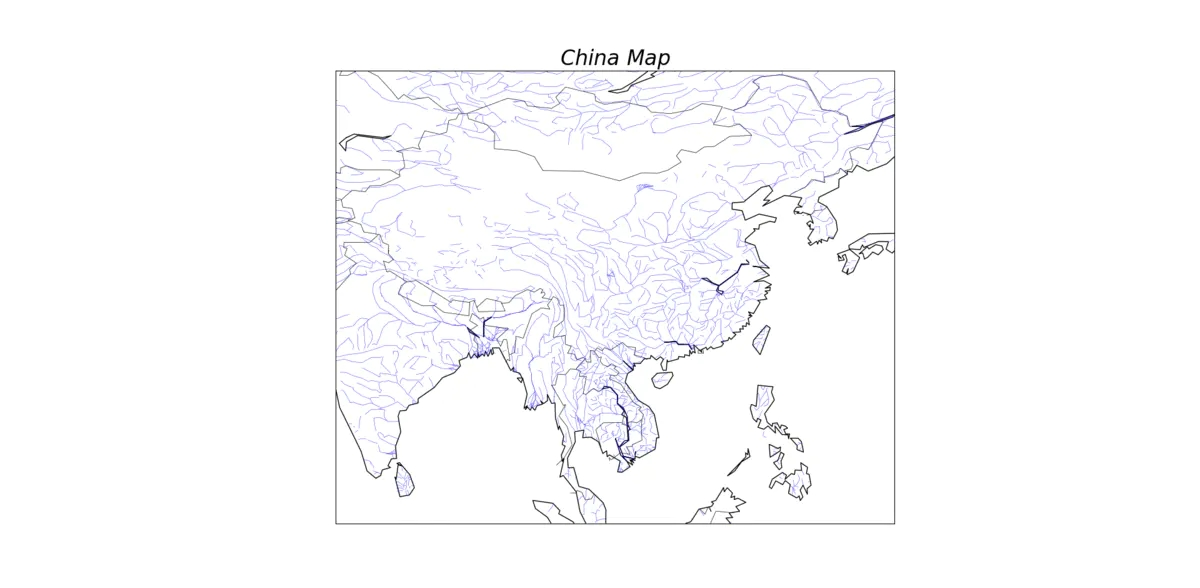好了，一个中国地图就出来了！但是我们发现，好像少了点什么，没错就是省界。我们可以在下载中国大陆和台湾省的行政区域的shape文件，下载后解压，然后加入下面的代码。chn='g:\python_material\mapofchina'chn的值就是解压后的地图文件所在的地址。下面我们就可以加入省界了！map.readshapefile(chn+'\gadm36_chn_shp\gadm36_chn_1','states',drawbounds=true)别忘了把台湾省加上去map.readshapefile(chn+'\gadm36_twn_shp\gadm36_twn_1','taiwan',drawbounds=true)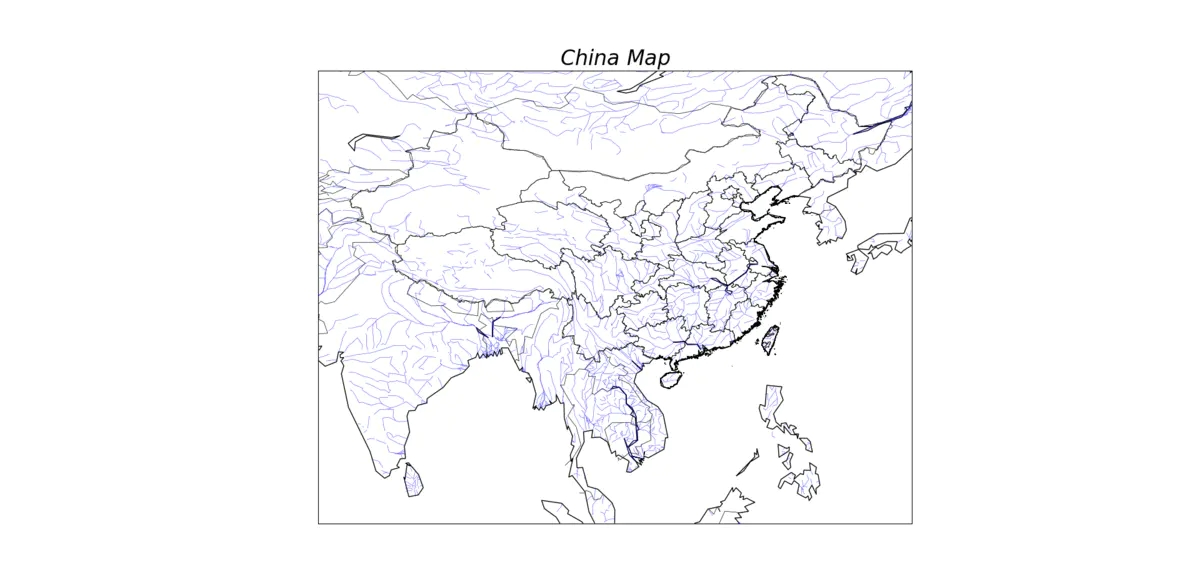还可以在地图上加上经纬度，比如我们要画5条经纬线，可以这么做：parallels = np.linspace(3,55,5)map.drawparallels(parallels,labels=[true,false,false,false])meridians = np.linspace(70,140,5)map.drawmeridians(meridians,labels=[false,false,false,true])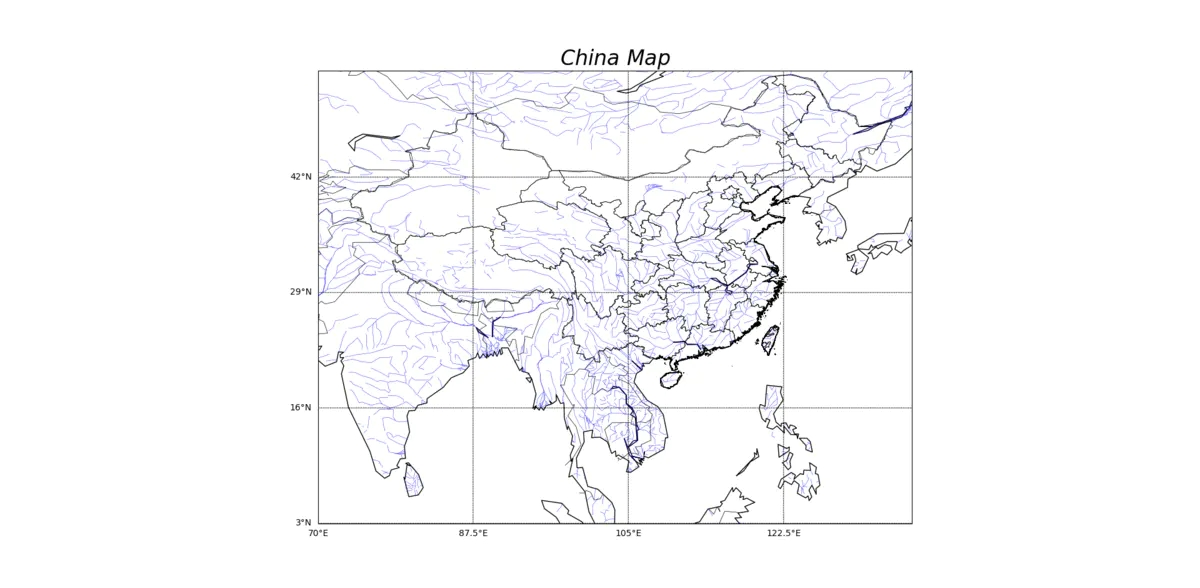大功告成！但是，emmm，我们发现好像有点歪？我们可以在创建地图时选择投影参数。map=basemap(llcrnrlon=70,llcrnrlat=3,urcrnrlon=137,urcrnrlat=54,projection = 'lcc', lat_1 = 33, lat_2 = 45, lon_0 = 100)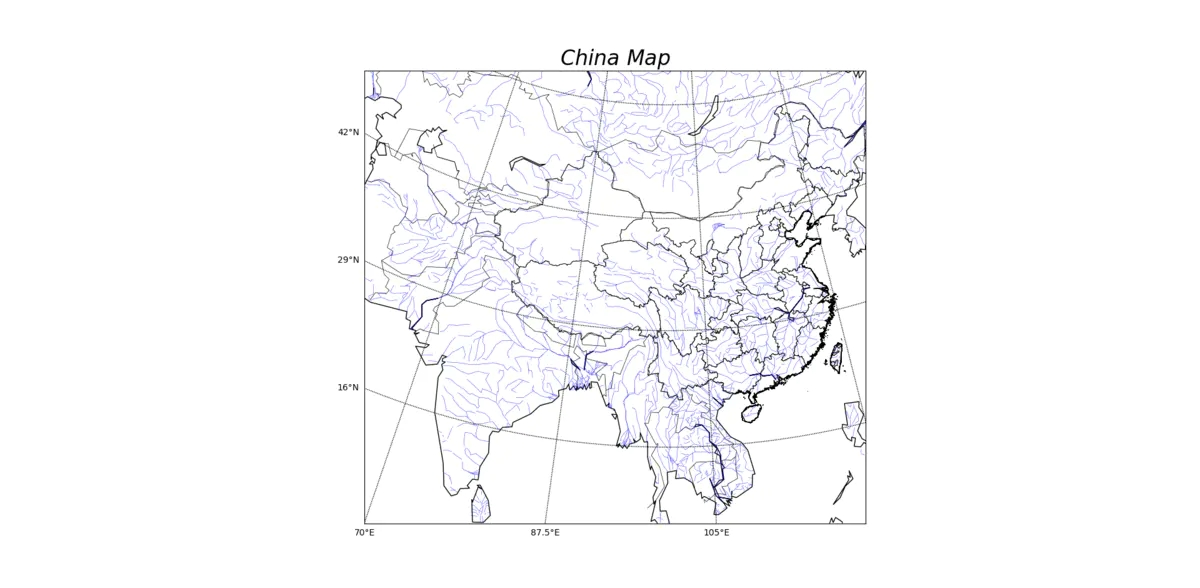这回正式的完成了！附上所有代码：import numpy as npimport matplotlib.pyplot as pltfrom mpl_toolkits.basemap import basemapplt.figure(1)map=basemap(llcrnrlon=70,llcrnrlat=3,urcrnrlon=139,urcrnrlat=54,projection = 'lcc', lat_1 = 33, lat_2 = 45, lon_0 = 100)map.drawcoastlines()map.drawcountries()map.drawrivers(color='blue',linewidth=0.3)chn='g:\python_material\mapofchina'map.readshapefile(chn+'\gadm36_chn_shp\gadm36_chn_1','states',drawbounds=true)map.readshapefile(chn+'\gadm36_twn_shp\gadm36_twn_1','taiwan',drawbounds=true)parallels = np.linspace(3,55,5)map.drawparallels(parallels,labels=[true,false,false,false])meridians = np.linspace(70,140,5)map.drawmeridians(meridians,labels=[false,false,false,true])plt.title(r'$china\ map$',fontsize=24)plt.show()以上就是利用python绘制中国地图的详细内容，更多关于python 绘制地图的资料请关注萬仟网其它相关文章！希望与广大网友互动？？点此进行留言吧！
展开全文• 大尺度地学研究需要绘制世界地图范围内的地理对象变化。网上很多python matplotlib资料，但很多函数用法都没解释清楚，例如颜色栏使用方法及位置管理等方法。当然一开始就使用GMT（Generic mapping tool）工具绘制...
大尺度地学研究需要绘制世界地图范围内的地理对象变化。网上很多python matplotlib资料，但很多函数用法都没解释清楚，例如颜色栏使用方法及位置管理等方法。当然一开始就使用GMT（Generic mapping tool）工具绘制地图的想法，证明就是一个彻底的弯路。本文介绍的方法对于绘制小区域变化信息也是适用的,与此同时它也是数据科学专业研究必备。会话多说，直接上代码和图片结果：import geopandas as gpd
import pandas as pd
import shapely.geometry
import matplotlib
matplotlib.use('Agg')
from shapely.geometry import Polygon
import json
from mpl_toolkits.basemap import Basemap
import plotly.graph_objects as go
from mpl_toolkits.axes_grid1 import make_axes_locatable
import matplotlib.pyplot as plt
import shapefile as shp
import seaborn as sns
import numpy as np
import matplotlib as mpl
sns.set(style="whitegrid", palette="pastel", color_codes=True)
sns.mpl.rc("figure", figsize=(10,6))

if __name__ == "__main__":
#serach a different projection of the basemap
m = Basemap(resolution='c',
projection='kav7',
lat_0=0., # Center around
lon_0=0.) # lat 0, lon 0
m.drawcoastlines()
m.fillcontinents(color='coral',lake_color='aqua')
# draw parallels and meridians.
m.drawparallels(np.arange(-90.,91.,30.))
m.drawmeridians(np.arange(-180.,181.,60.))
m.drawmapboundary(fill_color='aqua')
#plt.title("Mercator Projection")
n_graticules = 18.
parallels = np.arange(-80., 90, n_graticules)
meridians = np.arange(0., 360., n_graticules)
lw = 1
dashes = [5,7] # 5 dots, 7 spaces... repeat
graticules_color = 'grey'

fig1 = plt.figure(figsize=(16,20))
fig1.patch.set_facecolor('#e6e8ec')

m.drawmapboundary(color='white', linewidth=0.0, fill_color='white')
m.drawparallels(parallels, linewidth=lw, dashes=dashes, color=graticules_color)
m.drawmeridians(meridians, linewidth=lw, dashes=dashes, color=graticules_color)
m.drawcoastlines(linewidth=0)
m.fillcontinents('grey', lake_color='grey')
m.drawcountries(linewidth=1, linestyle='solid', color='white', zorder=30)

#title = plt.title('World map (Kavrayskiy 7)', fontsize=20)
#title.set_y(1.03) # Move the title a bit for niceness

#the second step is ploy symbols on the maps
#we should use matplotlib.pyplot.scatter function
#plt.savefig('thisarea.png', bbox_inches='tight')
#plot some shapefile on the basemap as symbols
#get the centriod points of thegeomerties
data.columns = ["LONGITUDE", "LATITUDE"]
#print(data)
lats = data['LATITUDE'].values
lons = data['LONGITUDE'].values
x1, y1 = m(lons, lats) # Convert coords to projected place in figur

cmap=plt.cm.jet
cmaplist = [cmap(i) for i in range(cmap.N)]
cmaplist = (.5, .5, .5, 1.0)
#in fact it's the RGBA
bounds = np.arange(0.5, 10.5, 1)
print(bounds)
norm = mpl.colors.BoundaryNorm(bounds, cmap.N)
print(norm)
tag = np.random.randint(1, 10, 68)
#we define our numerical value and store them in te tag
#tag[10:12] = 0
print(tag)
cmap = mpl.colors.LinearSegmentedColormap.from_list(
'Custom cmap', cmaplist, cmap.N)
#c represent marker color, s represent marker size
#if numerical value is provided to C as the tag,
m.scatter(x1, y1,
c=tag,
cmap=cmap, norm=norm,
marker="o",
zorder=10,
alpha=0.8)
#print(x1)
#we shoud define custom discrete colorbar in the third step
divider = make_axes_locatable(ax)
#ax2 = fig1.add_axes([0.95, 0.1, 0.03, 0.8])
cb = mpl.colorbar.ColorbarBase(cax, cmap=cmap, norm=norm,
spacing='proportional', boundaries=bounds, format='%1i')
cb.ax.set_yticklabels(['-100--50','-50--20','-20--10','-10-0','0-10','10-20','20-50', '50-100', '>100'])  # horizontal colorbar
cax.set_ylabel('Area change (%)')
#print(np.random.randint(100, 500, 68))
#modify ticks info
plt.savefig('thisarea.png', bbox_inches='tight')
#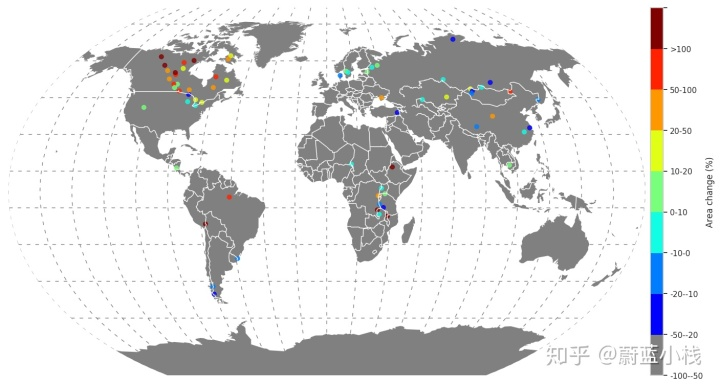这里的点表示的是世界上面积最大的一些代表性湖泊矢量中心点的经纬度坐标，然后记住需要转换到对应的投影面上。这里选择kav7投影，主要是它和wgs84比较接近，而且显示出来的效果比较好看一点。画图时最重要的是下面函数，看起来函数解释很全的，说白了就是给定x,y位置，在对应画板上画出指定大小和颜色的标记。A scatter plot ofyvs.xwith varying marker size and/or color.matplotlib.pyplot.scatter(x,y,s=None,c=None,marker=None,cmap=None,norm=None,vmin=None,vmax=None,alpha=None,linewidths=None,verts=<deprecated parameter>,edgecolors=None,*,plotnonfinite=False,data=None,**kwargs)但这里有几个函数参数要仔细解释一下：cmap：AColormapinstance or registered colormap name.cmap is only used ifc is an array of floats这里的解释非常具有误导性和表述模糊不清。实际上，正确的接应该是将它看成0与1之间的浮点数值与RGBA之间的对照表。我们的目标是将这些湖泊变化按照面积变化的几个刻度进行分类，然后通过匹配这些分类的颜色表，将其标注在世界地图上。例如这里的“-50--20”表示面积在一段时期变小-50-20%之间的湖泊用蓝色标注出来。For floats, X should be in the interval [0.0, 1.0] to return the RGBA values X*100 percent along the Colormap line.For integers, X should be in the interval [0, Colormap.N) to return RGBA values indexed from the Colormap with index X.这里存在的第一个问题是明明我要用9种颜色表示，为什么颜色栏和值不对应？实际上，我们需要定义两个变量来存储，然后想办法使用matplotlib.ticker.Formatter Norm: ,norm is used to scale the color data,c, in the range 0 to 1, in order to map into the colormapcmap （简单理解就是将颜色数据映射到0到1范围）之所以颜色栏和标注值不对应，主要是因为bounds 元素个数与set_yticklabels中自定义标签个数不对应，然后还要考虑设置ColorbarBase的ticks参数，让颜色值落在【0.9】之间    cmap=plt.cm.jet    cmaplist = [cmap(i) for i in range(cmap.N)]    cmaplist = (.5, .5, .5, 1.0)    #in fact it's the RGBAbounds = np.arange(0.5, 10.5, 1)    print(bounds)    print(bounds-0.5)    print("......")    norm = mpl.colors.BoundaryNorm(bounds, cmap.N)    print(norm)    tag = data['RESULTS'].values    #we define our numerical value and store them in te tag     #tag[10:12] = 0    print(tag)    s = [ i*100  for i in tag]    cmap = mpl.colors.LinearSegmentedColormap.from_list(    'Custom cmap', cmaplist, cmap.N)    #c represent marker color, s represent marker size     #if numerical value is provided to C as the tag,     m.scatter(x1, y1,          c=tag,          s = s,          cmap=cmap,           norm=norm,          marker="o",           zorder=10,           alpha=0.8)    #print(x1)    #we shoud define custom discrete colorbar in the third step    divider = make_axes_locatable(ax)    cax = divider.append_axes("right", size="2%", pad=0.1)    #ax2 = fig1.add_axes([0.95, 0.1, 0.03, 0.8])    cb = mpl.colorbar.ColorbarBase(cax, cmap=cmap, norm=norm,    spacing='proportional', ticks=bounds-0.5)    cb.ax.set_yticklabels(['-100--50','-50--20','-20--10','-10-0','0-10','10-20','20-50', '50-100', '>100'])  # horizontal colorbar    cax.set_ylabel('Area change (%)')    #print(np.random.randint(100, 500, 68))    #modify ticks info    plt.savefig('thisarea.png', bbox_inches='tight')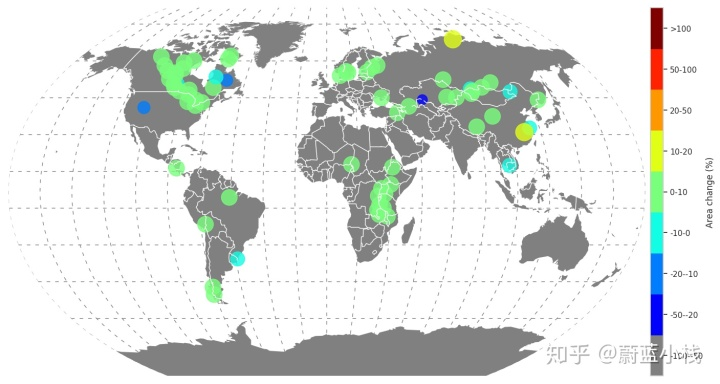此外，本文还使用了，相对轴域概念，在地图右边附加一个colorbar. 在后续，我们将添加如何在基本地图上添加连续变化数据集的可视化表示方法。谢谢大家持续关注我们    divider = make_axes_locatable(ax)    cax = divider.append_axes("right", size="2%", pad=0.1)
展开全文• 我们可以使用Basemap这个工具包来实现中国地图绘制首先需要加载一些包：import numpy as npimport matplotlib.pyplot as pltfrom mpl_toolkits.basemap import BasemapBasemap包就是气象画图的利器，现在我们就...
我们可以使用Basemap这个工具包来实现中国地图的绘制首先需要加载一些包：import numpy as npimport matplotlib.pyplot as pltfrom mpl_toolkits.basemap import BasemapBasemap包就是气象画图的利器，现在我们就可以愉快的画图了！plt.figure(1)map=Basemap()map.drawcoastlines()plt.title(r'$World\ Map$',fontsize=24)plt.show()第2行创建一个地图，第3行添加海岸线，这样一个世界地图就出来了，怎么样，很简单吧。(plt.show()这行代码是用来显示图片的)![wordmap_国界线.png](https://upload-images.jianshu.io/upload_images/15011972-506e6a107e6c56eb.png?imageMogr2/auto-orient/strip%7CimageView2/2/w/1240)我们发现这只是海岸线图，那么怎么将国界线添加上去呢？很简单，只要添加一行代码就可以了。map.drawcountries()wordmap_国界线.png那么怎么添加河流呢？可能有些同学已经猜到了，就是drawrivers()map.drawrivers(color='blue',linewidth=0.3)wordmap_河流.png好了，现在我们可以开始画中国地图了！其实只要在创建地图时指定一下范围就可以了，查阅资料发现，中国的经纬度范围是东经135度2分30秒-东经73度40分，北纬3度52分-北纬53度33分。map=Basemap(llcrnrlon=70,llcrnrlat=3,urcrnrlon=139,urcrnrlat=54)chinamap.png好了，一个中国地图就出来了！但是我们发现，好像少了点什么，没错就是省界。我们可以在https://gadm.org/download_country_v3.html下载中国大陆和台湾省的行政区域的shape文件，下载后解压，然后加入下面的代码。CHN='G:\python_material\MapOfChina'CHN的值就是解压后的地图文件所在的地址。下面我们就可以加入省界了！map.readshapefile(CHN+'\gadm36_CHN_shp\gadm36_CHN_1','states',drawbounds=True)别忘了把台湾省加上去map.readshapefile(CHN+'\gadm36_TWN_shp\gadm36_TWN_1','taiwan',drawbounds=True)chinamap_省界.png还可以在地图上加上经纬度，比如我们要画5条经纬线，可以这么做：parallels = np.linspace(3,55,5)map.drawparallels(parallels,labels=[True,False,False,False])meridians = np.linspace(70,140,5)map.drawmeridians(meridians,labels=[False,False,False,True])chinamap_省界,经纬线.png大功告成！但是，emmm，我们发现好像有点歪？我们可以在创建地图时选择投影参数。map=Basemap(llcrnrlon=70,llcrnrlat=3,urcrnrlon=137,urcrnrlat=54,projection = 'lcc', lat_1 = 33, lat_2 = 45, lon_0 = 100)chinamap_省界,经纬线,投影.png这回正式的完成了！附上所有代码：import numpy as npimport matplotlib.pyplot as pltfrom mpl_toolkits.basemap import Basemapplt.figure(1)map=Basemap(llcrnrlon=70,llcrnrlat=3,urcrnrlon=139,urcrnrlat=54,projection = 'lcc', lat_1 = 33, lat_2 = 45, lon_0 = 100)map.drawcoastlines()map.drawcountries()map.drawrivers(color='blue',linewidth=0.3)CHN='G:\python_material\MapOfChina'map.readshapefile(CHN+'\gadm36_CHN_shp\gadm36_CHN_1','states',drawbounds=True)map.readshapefile(CHN+'\gadm36_TWN_shp\gadm36_TWN_1','taiwan',drawbounds=True)parallels = np.linspace(3,55,5)map.drawparallels(parallels,labels=[True,False,False,False])meridians = np.linspace(70,140,5)map.drawmeridians(meridians,labels=[False,False,False,True])plt.title(r'$China\ Map$',fontsize=24)plt.show()
展开全文• powerworldGIS绘制着色的中国地图信息（省、河流、地区等）
•  //地图   int playerPos1; //对战中玩家1的当前位置   int playerPos2; //对战中玩家2的当前位置  String[] goAndStop = new String; //走或停标识设置  String[] playerName = new ...联想 graph string class
• 用于GIS制图中中国地图绘制地图，含河流、湖泊等信息多个图层
• 北京shp文件（cpg/dbf)包含铁路河流大厦建筑地点 gis/r地图文件 方便绘制地图 谢谢下载
• 但是，对于小白来说地图绘制却不得要领 基于此，MC软件增加此功能，结合Echarts，一键绘制地域分布图 操作步骤与主题河流图一致， 第一步：打开软件，输入“中国地图”，点击转化 第二步：将点击中国地图，将转化...
• 官方公开的数据包括：地图数据，及居住地、交通、河流等辅助数据。地图数据有4个压缩文件：bou1_4m.zip、bou2_4m.zip、bou3_4m.zip和bou4_4m.zip。bou代表边界的意思，数字1~4代表国家、省、市、县的4级行政划分；4m...
• 对于环境建筑设计和规划专业的小伙伴来说，各种前期分析是最让人头疼的，其中底图的获取和绘制是非常重要的一步，明确、美观、详细的底图是极其重要的。 -- 文末获取：百度地图数据一键爬取神器+安装教程--得益于...
• 在实际的应用中，线对象主要用来描述线状地物，如河流、铁路、道路、电力线等。应用程序也提供了多种绘制折线的方式，满足不同应用情景下的绘制要求。直线绘制直线在“对象操作”选项卡的“对象绘制”组中，单击“线...
• 学习目标与要求： 1．复习python读取json文件； 2．掌握pyecharts绘制树图、矩形...掌握地理热力图、地图上标注点的绘制，理解地理/地图数据可视化的含义。 下面根据几个例题来介绍： 1、使用以下JSON数据绘制树图、
• mapbox 先上效果图 再来一段官网描述：Mapbox GL JS 是一个 JavaScript 库，它使用 WebGL，以 vector tiles 和 Mapbox styles ...包括平常的河流、土地、天空等颜色，还有各种建筑是否显示地区名字、中英文等。 代码 #js
• 做毕设其中需要一个功能就是使用笔刷在地图绘制河流之类的东西。如果只是绘制贴图或者顶点颜色，得到一张图片还是不难实现的。但是根据我后面功能的需求，我需要得到的是一个多边形顶点的数据，而不是一张图片。...unity 多边形 polygon 布尔运算
• CorelDraw地图制图讲义，可帮助学习。包含河流绘制、行政区划绘制、交通线绘制、各种符号绘教程。
• 本套世界地图shape文件底图以国家测绘地理信息局公布的世界标准地图（编号：GS（2016）1666）为参考绘制，用该底图已投两个C刊，均符合期刊图形审查要求！底图是最新2019版的，有详细和简单两个版本，简单版包含国境...
• 作者:鲤鱼QQ：2952930492【插件预览】【插件介绍】GEOlayers 3 使您可以直接在After Effects中设计和设置地图动画。您可以轻松地将建筑物绘制到After Effects形状图层上，突出显示国家边界，街道，湖泊，河流，地方...
• 著名的古代巴比伦地图是原始地图中最具有代表性的作品之一，它是公元前2500年的作品，绘制在黏土片上，绘有山岭，城镇，河流 比利时考古学家在意大利南部出土陶器残片。公元前500年左右，南部普利亚地区西方文明最早...
• 本套世界地图shape文件底图以国家测绘地理信息局公布的世界标准地图（编号：GS（2016）1666）为参考绘制，用该底图已投两个C刊，均符合期刊图形审查要求！底图是最新2019版的，有详细和简单两个版本，简单版包含国境...
• 一系列的地理要素(例如一系列的河流)，而每个要素都有自己的属性(例如每个河流都有自己的长度)，为了方便对地理数据的操作，MATLAB将数据打包成地理数据结构体(geographic data structures)。一个地理数据结构体是一...
• OSM的地图由用户根据手提GPS装置、航空摄影照片、其他自由内容甚至单靠地方智慧绘制。网站里的地图图像及向量数据皆以共享创意姓名标示-相同方式分享2.0授权。 请注意虽然信息非常全，但是这个地图并不包含台湾省，...
• OSM的地图由用户根据手提GPS装置、航空摄影照片、其他自由内容甚至单靠地方智慧绘制。网站里的地图图像及向量数据皆以共享创意姓名标示-相同方式分享2.0授权。 请注意虽然信息非常全，但是这个地图并不包含台湾省，...
• 可以在图上进行处理，方便进行研究，含有主要河流，城市中心点位，包括长江，是画图的一个很好的工具，可以用来进行绘制所需要的点位图
• ## matlab map world.shp

热门讨论 2013-02-02 02:28:18
matlab 绘制中国地图 绘制世界地图 河流 铁路